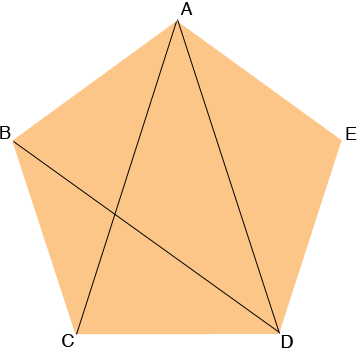SEARCH HOMEMath Central Quandaries & QueriesQuestion from Jerry, a student: All five angles of a pentagon ABCDE are equal. The diagonals AC, AD and BD have the same length. Prove that all five sides of the pentagon are equal in length.Hi Jerry,Triangle ACD is isosceles so angles ACD and CDA have the same measure. Thus angles BCA and ADE have the same measure. Thus triangles ABC and ADE are similar and since |AC| = |AD| triangles ABC and ADE are congruent.

Repeat this argument using the fact that triangle ADB is isosceles.

PennyMath Central is supported by the University of Regina and The Pacific Institute for the Mathematical Sciences.